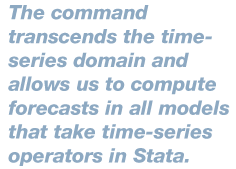Home  /  Stata News  /  Vol 29 No 3 (2014 quarter 3)  /  forecast## In the spotlight: forecast for dynamic panel data and counterfactuals

The new forecast command in Stata 13 allows us to use estimates from models with time-varying components. The command transcends the time-series domain and allows us to compute forecasts in all models that take time-series operators in Stata. For instance, we can use it to compute forecasts after reg3 or after panel-data estimation. In the [TS] Time-Series Reference Manual, there is an example using reg3. I will illustrate an example using dynamic panel-data models.I have data for 100 individuals from 2003 to 2010 for country A. I will explore how an increase of 10% in its minimum wage for the entire sample period would have affected people's income and their employment probability, a counterfactual. There are two underlying assumptions: first, that an increase in minimum wage will increase individual income for people who earn the minimum wage and for those whose remuneration, contractually, is a multiple of the minimum; and second, that, at the same time, an increase in minimum wage will decrease the probability of employment. We would like to see which of these effects dominates once we increase the minimum wage.I will estimate three models. The first one models the probability of employment conditional on the current minimum wage (mwage), if GDP is below its potential (belowgdp), the individual's years of schooling (education), whether the individual belongs to a union (union), the individual's gender (gender), whether the individual is married (married), and the interaction between marital status and gender (married#gender). The second one models wages conditional on wages from the previous period, the current level of the minimum wage, the years of schooling of the individual, age, and the interaction between married and gender.

The third model reflects the fact that the minimum wage is fixed every year in negotiations between the government and the union representatives. Thus, the minimum wage is explained mostly by the minimum wage from last period and by unobservable idiosyncratic shocks.

Below, I fit the aforementioned models and store the results:

. xtabond wage education mwage age
. estimates store wage
. xtprobit employed married#gender gender married belowgdp education union mwage, pa
. estimates store employed
. xtabond mwage
. estimates store mwage


I will now use these estimates to create two forecasts—one with the current data and the other where the only variable that changes is the wage (by 10% every period).

I call this forecast wages and use the forecast create command. I then tell forecast that I want to add the results from the three estimates above by using the forecast estimates command as follows:

. forecast create wages, replace
. forecast estimates wage
. forecast estimates employed
. forecast estimates mwage


I now solve the forecast for the three endogenous variables in our model by using the forecast solve command. This generates three new variables, f_employed, f_wage, and f_mwage, where the f_ prefix is the forecast default name for the generated forecasts.

. forecast solve, begin(2008)

Computing dynamic forecasts for model wages.

Starting period:  2008
Ending period:    2010
Number of panels: 100
Forecast prefix:  f_
(output omitted)


Now, I construct the counterfactual scenario where the 10% minimum-wage increase occurred, and I use the prefix new_ to distinguish the result from the previous case. As before, I do this by using forecast solve and forecast adjust for my counterfactual.

. forecast adjust mwage = mwage*1.1
Endogenous variable mwage now has 1 adjustment.

. forecast solve, begin(2008) prefix(new_)

Computing dynamic forecasts for model wages.

Starting period:  2008
Ending period:    2010
Number of panels: 100
Forecast prefix:  new_


If we look at the average values in both scenarios, we see the following:

. mean f_wage new_wage f_employed new_employed

Mean estimation                     Number of obs    =     800

Mean   Std. Err.     [95% Conf. Interval]

f_wage     90663.82     1501.7      87716.07    93611.56
new_wage     90973.68   1512.479      88004.78    93942.58
f_employed      .932823   .0080659      .9169902    .9486557
new_employed     .9184451   .0084635      .9018318    .9350584


Thus, wages increase by about 300 units of the currency of country A, and there is a decrease in the probability of being employed of more than 1 percentage point on average. Therefore, in this case, the effect on employment seems more substantial than the increase of income.

Of course, this is a hypothetical research exercise, but it helps to illustrate that you can use forecast beyond the time-series spectrum and to construct counterfactual exercises.

—Enrique Pinzon
Senior Econometrician